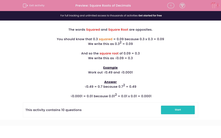# Square Roots of Decimals

In this worksheet, students state the square roots of decimal numbers.Key stage:  KS 2

Curriculum topic:   Maths and Numerical Reasoning

Curriculum subtopic:   Decimals

Difficulty level:#### Worksheet Overview

The words Squared and Square Root are opposites.

You should know that 0.3 squared = 0.09 because 0.3 x 0.3 = 0.09

We write this as 0.32 = 0.09

And so the square root of 0.09 = 0.3

We write this as √0.09 = 0.3

Example

Work out √0.49 and √0.0001

√0.49 = 0.7 because 0.72 = 0.49

√0.0001 = 0.01 because 0.01= 0.01 x 0.01 = 0.0001

### What is EdPlace?

We're your National Curriculum aligned online education content provider helping each child succeed in English, maths and science from year 1 to GCSE. With an EdPlace account you’ll be able to track and measure progress, helping each child achieve their best. We build confidence and attainment by personalising each child’s learning at a level that suits them.

Get started